Latest Teaching jobs   »   Congruence And Similarity Of Triangles :...

# Congruence And Similarity Of Triangles : Download Maths Notes For CTET Exam Free PDFMathematics is an equally important section for CTET, MPTET, KVS & DSSSB Exams and has even more abundant importance in some other exams conducted by central or state govt. Generally, there are questions asked related to basic concepts and Facts of the Congruence And Similarity Of Triangles.

To let you make the most of Mathematics section, we are providing important facts related to the Congruence And Similarity Of Triangles. At least 2-3 questions are asked from this topic in most of the teaching exams. We wish you all the best of luck to come over the fear of the Mathematics section.

How to Overcome Exam Fever, Especially When You Fear Maths

## Congruence and Similarity of Triangles

Congruent Triangles: Triangles are congruent when they have exactly the same three sides and exactly the same three angles.

What is “Congruent”?

It means that one shape can become another using turns, flips and/or sliders.

The equal sides and angles may not be in the same position (if there is a turn or a flip), but they are there.

Same Sides: When the sides are same then the triangles are congruent.

For example: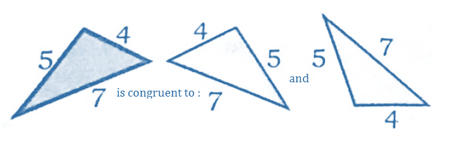Same Angles: Two triangles with the same angles might be congruent.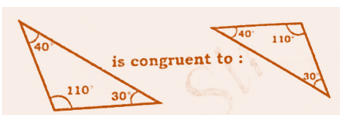1. SSS congruent

Side – Side – Side congruence. When two triangles have corresponding sides equal that are congruent as shows below, the triangles are congruent.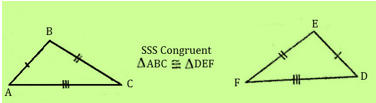1. SAS Congruence

Side – Angle – Side Congruence. When two triangles have corresponding angles and sides equal that are congruent as shown below, the triangles are congruent.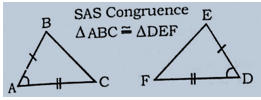1. ASA Congruence

Angle – Side – Angle Congruence. When two triangles have corresponding angles and sides equal that are congruent as shown below, the triangles themselves are congruent.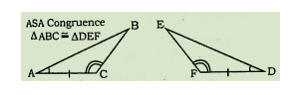Mathematics Study Notes For All Teaching Exams

1. AAS Congruence OR SAA Congruence

Angle – Angle – Side congruence. When two triangles have corresponding angles and sides equal that are congruent as shown below, the triangles are congruent.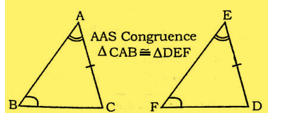1. HL Congruence

Hypotenuse – leg congruence. When two triangles have corresponding sides equal that are congruent as shown below, the triangles are congruent.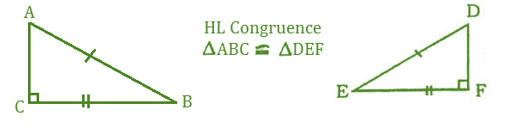Similarity of Triangles

Two triangles are similar if and only if the corresponding sides are in proportion and the corresponding angles are congruent.

How To Score 25+ Marks In Mathematics For CTET Exam?

Methods of proving triangles similar:

• If two angles of one triangle are congruent to two angles of another triangle, the triangles are similar.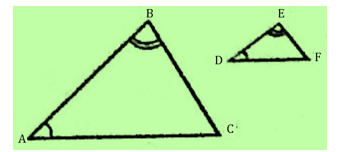If ∠ A = ∠ D

∠ B = ∠ E

Then : ∆ ABC ∼ ∆ DEF

• If the three sets of corresponding sides of two triangle are in proportion, the triangles are similar.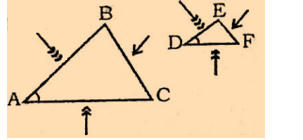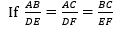Then : ∆ ABC ∼ ∆ DEF

Practice More Mathematics  Quiz Here

• If an angle of one triangle is congruent to the corresponding angle of another triangle and the lengths of the sides including these angles are in proportion, the triangles are similar.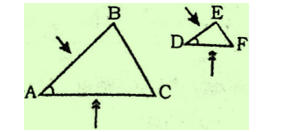If  ∠ A = ∠ D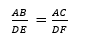Then : ∆ ABC ∼ ∆ DEF

• If a line is parallel to one side of a triangle and intersects the other two sides of the triangle, the line divides these two sides proportionally.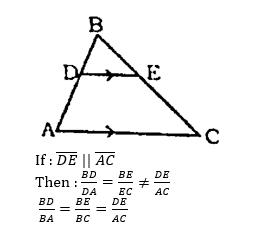Properties of Similar triangles

If the two triangles are similar, then for the proportional / corresponding sides have the following results.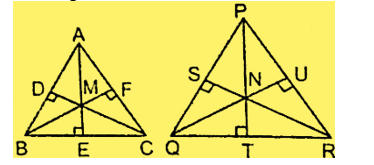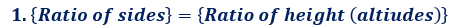= Ratio of medians

= Ratio of angles bisectors

= Ratio of inradii

= Ratio of circumradii

1. Ratio of areas = Ratio of squares of corresponding sides.

i.e. If ∆ ABC ∼ ∆ PQR,

Then,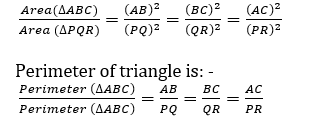1. In a right-angled triangle, the triangles on each side of the altitude drawn from the vertex of the right angle to the hypotenuse are similar to the original triangle and to each other too.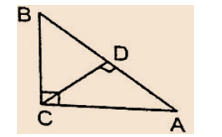i.e., ∆ ABC ∼ ∆ BDC ∼∆ CDA.

### Some facts on Right angle triangle

• CD² = BD × DA
• BC × CA = BA × CD
• BC² = BD × BA
• AC² = AD × BA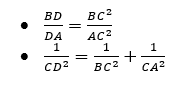Area Based question when two triangles are similar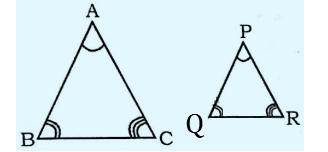If ∆ ABC ∼ ∆ DEF Then

The Ratio of their area is the square of their sides, Medians, Altitudes, Perimeters, Angle Bisectors.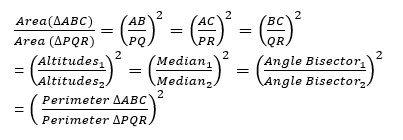•विशेषण- परिभाष�...
•Phases of Teaching - Stages of Teaching ...
•Operant and Classical Conditioning - Dif...
•संज्ञा: परिभाष�...
•अलंकार - परिभाष�...
•Stages of Development- Child Growth and ...